Thermal Deformation and Dynamic Recrystallization of 17-4PH Stainless Steel.(HY-industry technical centre)

## Test materials and procedures

### Table 1 shows the chemical composition of the 17-4 PH stainless steel used in this research. The sample used for the thermal compression test was a circular sample with a diameter of 5 mm and a height of 10 mm. The sample was in the shape of Rastegaev, and glass frit was used as a lubricating material for the contact surface of the drill seat and the sample. A Baehr DIL-805 thermal expansion instrument was used to perform the thermal compression test. Prior to the start of the test, the samples were austenitized at a temperature of 1180 ° C for 10 minutes, and then held for 5 minutes. After this process, the size of austenite grains is D0 = 105 μm (see Figure 1 (a)). Then, under a true strain of about 0.9, a single thermal compression test was performed at a temperature of 950 to 1150 ° C at a strain rate of 10-3 to 10 s-1.### After removing the elastic part, each rheological curve is corrected for friction, and then smoothing is performed to eliminate the bumps and undulations as described below. Using a suitable lubricant in a thermal compression test can significantly reduce friction, but it cannot completely eliminate friction. Therefore, in order to form friction corrected data, the influence of friction should be considered in the original stress data. Ebrahimi and Najafizadeh proposed a simplified theoretical analysis of the drum compression test for determining the friction constant (m) based on the upper bound theory. The required expression is as follows: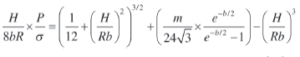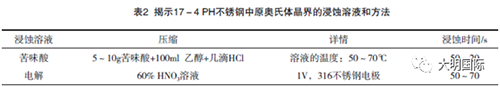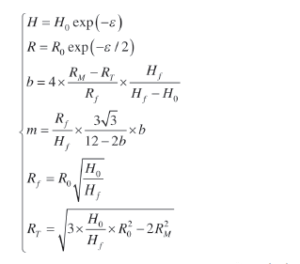## 3. Results and discussion

### The figure shows that, regardless of the shape of the rheological curve, the sample has obvious inflection points (related to the minimum value of the -dθ / dσ-σ curve shown in Figure 4 (b)), which is possible. Related to the emergence. Figure 4 (c) shows the microstructure of this sample. In this microstructure, some fine grains can be seen at the serrated grain boundaries, which proves that DRX occurs in this case. For the deformation conditions of T = 1000 ° C and ε = 10s-1, the shape of the rheological curve is similar to the typical DRX behavior (Figure 3). In the θ-σ curve (Figure 4 (d)) and -dθ / dσ-σ curve (Figure 4 ( e)), neither a clear inflection point nor a minimum value can be determined. As shown in Figure 4 (f), the softening outside the peak may be attributed to the adiabatic deformation temperature rise at 10 s-1. Flat grain boundaries in the microstructure of the sample also meant that no DRX was present.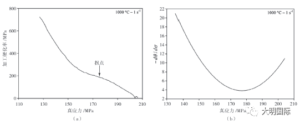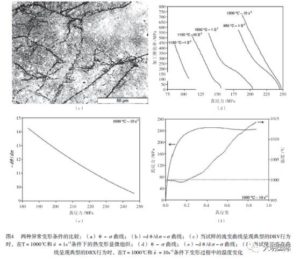### As shown by Sellars and Tegart using the hyperbolic sine function proposed by Garofalo, hot working can be viewed as a thermally activated process and can be described using a strain rate equation similar to that used in creep studies. According to these studies, the Zener-Hollomon parameter (Z), that is, the temperature-compensated strain rate, can be related to different types of flow stress (formula (4)): the power law at relatively low stress, and the Exponential law and hyperbolic sine law for various deformation conditions: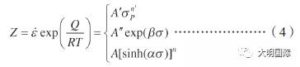### In many studies, one of the expressions of formula (6) comes from the literature that calculates the apparent activation energy. As the analysis above shows, the calculated values are very different. The deformation activation energy of 337 kJ / mol deviates from the diffusion activation energy in austenite, which is 280 kJ / mol. Although the activation energy of hot working depends on the material, it usually refers to the apparent value, because the internal microstructure state and the curve generated only from the experimental data are usually not considered, and the microstructure is assumed to maintain a constant value 3 3 7 k J / mol is used to calculate the Z parameter. According to formula (5),### the appropriate values of n and A can be obtained by using the curves of lnZ and ln {sinh (ασP)} (Fig. 6 (c)). These analyses lead to the following constitutive equations:### 3.4 Thermal deformation microstructure of 17-4PH Stainless Steel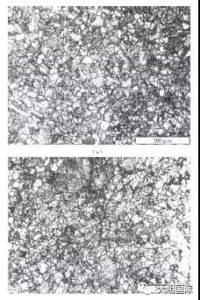### Figure 9 shows the microstructure of thermal deformation under different strain rate conditions when the deformation temperature is 1150 ° C for 17-4PH Stainless Steel. The figure shows that the average grain size increases with decreasing strain rate.